Go Math Grade 3 Chapter 8 Answer Key Pdf: contains all the topics which help the students to score better marks in the exams. The main aim of providing the Go Math Answer Key for Grade 3 Chapter 8 is to make the students understand the concepts in an easy manner. Download Go Math Grade 3 Answer Key Understand Fractions pdf. So, the students who are preparing for 3rd standard exams can check HMH Go Math Solution Key Grade 3 Chapter 8 Understand Fractions.

Check out the overview of Grade 3 HMH Go Math Solutions Key for Chapter 8 Understand Fractions here. So, make use of Answer Keys of Go Math 3rd Std Chapter 8 Understand Fractions and prepare well for your exams. You can find the practice questions at the end of the chapter.

Lesson 1: Equal Parts of a Whole

Lesson 2: Equal Shares

Lesson 3: Unit Fractions of a Whole

Lesson 4: Fractions of a Whole

Lesson 5: Fractions on a Number Line

Chapter 8 Mid-Chapter Checkpoint

Lesson 6: Relate Fractions and Whole Numbers

Lesson 7: Fractions of a Group

Lesson 8: Find Part of a Group Using Unit Fractions

Lesson 9: Problem Solving

Chapter 8 Understand Fractions Review/Test

Equal Parts for a Whole – Page No 447

Write the number of equal parts. Then write the name for the parts.

Question 1:Equal Parts: ___________
Name: _______________

i) 4
ii) Fourths

Explanation:
From the above figure, we can see that the circle is divided into 4 equal parts and the parts are named as fourths.

Question 2: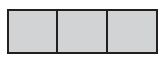Equal Parts: ___________
Name: _______________

i) 3
ii) Thirds

Explanation:
The rectangle is divided into 3 equal parts. The name for those parts is thirds.

Question 3: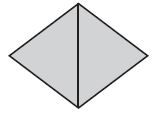Equal Parts: ____________
Name: ________________

i) 2
ii) Halves

Explanation:
The square is diagonally cut into 2 triangles. As it is a square the triangles will be of the same size. Therefore the triangles are equal and the name for the parts is halves.

Question 4: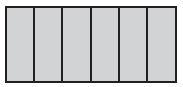Equal Parts: _____________
Name: _________________

i) 6
ii) Sixths

Explanation:
Here we can see a rectangle that is separated into 6 equal parts. As it is divided into 6 parts it is named as sixths.

Write whether the shape is divided into equal parts or unequal parts

Question 5: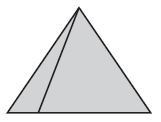____________ Parts

Unequal

Explanation:
The triangle is cut into 2 but the size and shape are not the same. So, by seeing the figure we can say that the parts are unequal.

Question 6: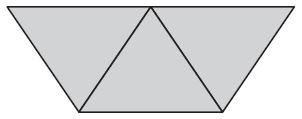____________ Parts

Equal

Explanation:
In the above figure, we can observe that the trapezium is divided into 3 triangles of equal parts.

Problem Solving

Question 7:
Diego cuts a round pizza into eight equal slices. What is the name for the parts?
____________

Eighths

Explanation:
Diego cuts a round pizza into 8 equal slices. So the name for the parts is eighths.

Question 8:
Madison is making a placemat. She divides it into 6 equal parts to color. What is the name for the parts?____________

Sixths

Explanation:
If the placemat is cut into 6 equal parts then the parts are named as sixths.

Equal Parts of a Whole – Lesson Check – Page No 448

Question 1:
How many equal parts are in this shape?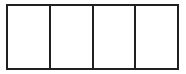Options:
i. 3
ii. 4
iii. 5
iv. 6

ii (4)

Explanation:
In the figure, the rectangle is divided into 4 equal parts.

Question 2:
What is the name for the equal parts of the whole?Options:
i. Fourths
ii. Sixths
iii. Eighths
iv. Thirds

iii (Eighths)

Explanation:
From the above fig, we can see 8 triangles. Therefore the name for the parts is eighths.

Spiral Review

Question 3:
Use a related multiplication fact to find the quotient.
49 ÷ 7 = ___

Options:
i. 6
ii. 7
iii. 8
iv. 9

ii (7)

Explanation:
Given,
49 ÷ 7 = ___
49/7 = 7
49 is divisible by 7 by 7 times. Therefore the remainder is 0 and the quotient is 7.

Question 4:
Find the unknown factor and quotient.
9 × __ = 45
Options:
i. 4
ii. 5
iii. 6
iv. 7

ii (5)

Explanation:
Given,
9 × __ = 45
45/9 = 5
Therefore the unknown factor of 9 × __ = 45 is 5.

Question 5:
There are 5 pairs of socks in one package. Matt buys 3 packages of socks. How many pairs of socks in all does Matt buy?
Options:
i. 30
ii. 15
iii. 10
iv. 8

ii (15)

Explanation:
Given that, there are 5 pairs of socks in 1 package.
If Matt buys 3 packages of socks then multiply 5 with 3. We get 15.
Therefore, Matt buys 15 pairs of socks.

Question 6:
Mrs. McCarr buys 9 packages of markers for an art project. Each package has 10 markers. How many markers in all does Mrs. McCarr buy?
Options:
i. 10
ii. 19
iii. 81
iv. 90

iv (90)

Explanation:
Given,
Mrs. McCarr buys 9 packages of marks for an art project
There are 10 markers in each package.

To find:
How many markers did Mrs. McCarr buy
In order to know the markers she bought we need to multiply the number of packages with total number of markers in each package.
i.e, 9 × 10 = 90
Therefore, the total number of markers in 9 packages is 90.

Equal Shares – Page No 453

Question 1:
6 friends share 3 sandwiches equally.3 Sixths of a Sandwich

Explanation:
There are 3 sandwiches and 6 friends need to share it equally
So, divide the total number of sandwiches by number of friends i.e., 3/6 = 1/2
So, the equal share of 6 sandwiches is 3 sixths or 1 half of a sandwich.

Question 2:
8 classmates share 4 pizzas equally.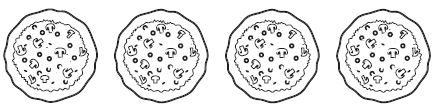4 Eighths, or 1 half of a pizza.

Explanation:
Given,
Number of pizzas = 4
Number of classmates = 8
In order to share the pizzas equally, we need to divide 4 by 8
4/8 = 1/2
So, the 8 classmates can share 4 eighths or 1 half of a pizza.

Question 3:
4 teammates share 5 granola bars equally. Draw to show how much each person gets. Shade the amount that one person gets. Write the answer.5 fourths of a granola bar.

Explanation:
Given that, the number of teammates = 4
and number of granola bars = 5
Number of granola bars/Number of teammates= 5/4
So, the answer is 5 fourths of a granola bars.

Problem Solving

Question 4:
Three brothers share 2 sandwiches equally. How much of a sandwich does each brother get?

2 thirds of a sandwich

Explanation:
To know how much sandwich does each brother get,
we have to divide no. of sandwiches by no. of brothers
i.e, 2/3
So, each brother gets 2 thirds of a sandwich.

Question 5:
Six neighbors share 4 pies equally. How much of a pie does each neighbor get?

4 sixths or 1 sixth or 2 thirds of a pie

Explanation:
Given that, 6 neighbors share 4 pies equally
To know how much of a pie does each neighbor get
we need to divide number of pies by number of neighbors
4/6 = 2/3

(or)

1/6 of each pie

Equal Shares Lesson Check Page No 454

Question 1:
Two friends share 3 fruit bars equally. How much does each friend get?Options:
i. 1 Half
ii. 2 Thirds
iii. 2 Halves
iv. 3 Halves

iv (3 Halves)

Explanation:
Total number of fruit bars/ Number of friends = 3/2
So, each friend gets 3 halves of the fruit bar

Question 2:
Four brothers share 3 pizzas equally. How much of a pizza does each brother get?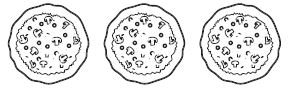Options:
i. 3 halves
ii. 4 third
iii. 3 fourths
iv. 2 fourths

iii. (3 fourths)

Explanation:
Given,
No. of pizzas = 3
No. of brothers = 4
In order to share the pizzas,
we need to divide No. of pizzas/No. of brothers = 3/4
So, the answer is 3 fourths.

Spiral Review

Question 3:
Find the quotient.
3)27
Options:
i. 6
ii. 7
iii. 7
iv. 9

iv (9)

Explanation:
To find quotient:
Divide 27/3 = 9
Therefore the quotient of 3)27 is 9

Question 4:
Tyrice put 4 cookies in each of 7 bags. How many cookies in all did he put in the bags?
Options:
i. 11
ii. 28
iii. 32
iv. 40

ii (28)

Explanation:
Given that, Tyrice put 4 cookies in each of 7 bags
Total no. of cookies = 4
Total no. of bags = 7
To find:
How many cookies in all did he put in the bags,
We need to multiply No. of bags with No. of cookies
we get 7 × 4 = 28
Therefore, the Total Number of cookies in all the bags is 28.

Question 5:
Ryan earns $5 per hour raking leaves. He earned$35. How many hours did he rake leaves?
Options:
i. 5 hours
ii. 6 hours
iii. 7 hours
iv. 35 hours

iii (7 hours)

Explanation:
Given,
Ryan earns $5 per hour To find how many hours did he rake leaves to earn$35
Divide 35 by 5, we get
35/5 = 7
So, Ryan raked leaves for 7 hours to earn $35 Question 6: Hannah has 229 horse stickers and 164 kitten stickers. How many more horse stickers than kitten stickers does Hannah have? Options: i. 45 ii. 65 iii. 145 iv. 293 Answer: ii (65) Explanation: Total no. of horse stickers Hannah has is 229 No. of kitten stickers Hannah has is 164 To know how many more horse stickers than kitten stickers does Hannah have, we need to subtract no. of horse stickers and no. of kitten stickers i.e., 229 – 164 = 65 So, the answer is 65 Unit Fractions of a Whole Page No 459 Write the number of equal parts in the whole. Then write the fraction that names the shaded part. Question 1: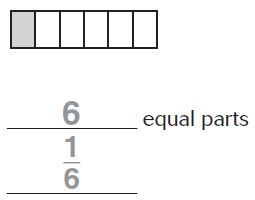__________ equal parts __________ Answer: i) 6 ii) 1/6 Explanation: The rectangle is divided into 6 equal parts. From the figure, we observe that one block is shaded. So, the fraction name of the shaded part is 1/6. Question 2: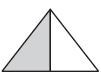__________ equal parts __________ Answer: i) 2 ii) 1/2 Explanation: There are 2 right-angled triangles in which one part is shaded. Therefore, the fractional name for the shaded part is 1/2 Question 3:__________ equal parts __________ Answer: i) 4 ii) 1/4 Explanation: The circle is divided into 4 equal parts and one part is shaded among them. The fraction that names the shaded part is 1/4 Question 4: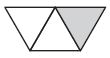__________ equal parts __________ Answer: i) 3 ii) 1/3 Explanation: In the above fig, we see that the trapezium is divided into 3 equal triangles and the fraction name of the shaded part is 1/3. Question 5: 1/3 isAnswer: Question 6: 1/8 is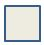Answer: Question 7: Tyler made a pan of cornbread. He cut it into 8 equal pieces and ate 1 piece. What fraction of the cornbread did Tyler eat? ________ Answer: 1/8 Explanation: There are 8 pieces of cornbread. Tyler ate 1 piece out of 8 cornbread. The fraction of cornbread that Tyler ate is 1/8 Question 8: Anna cut an apple into 4 equal pieces. She gave 1 piece to her sister. What fraction of the apple did Anna give to her sister? ________ Answer: 1/4 Explanation: Anna cut an apple into 4 pieces. She gave 1 piece of apple to her sister. One piece of apple/ Total Number of pieces = 1/4 Unit Fractions of a Whole Lesson Check Page No 460 Question 1: What fraction names the shaded part?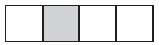Options: i. 1/3 ii. 1/4 iii. 1/6 iv. 1/8 Answer: ii (1/4) Explanation: There are 4 blocks in the rectangle and one part is shaded among them. So the answer is 1/4 Question 2: Tasha cut a fruit bar into 3 equal parts. She ate 1 part. What fraction of the fruit bar did Tasha eat? Options: i. 1/2 ii. 1/3 iii. 1/4 iv. 1/6 Answer: ii (1/3) Explanation: The fruit bar is cut into 3 equal parts and Tasha ate one part. So, the fraction name of the fruit bar that Tasha ate is 1/3 Spiral Review Question 3: Alex has 5 lizards. He divides them equally among 5 cages. How many lizards do Alex put in each cage? i. 0 ii. 1 iii. 5 iv. 10 Answer: ii (1) Explanation: Alex has 5 lizards and put them equally in 5 cages. That means Alex has put one lizard in one cage. Question 4: Find the product. 8 × 1 = _ i. 0 ii. 1 iii. 8 iv. 9 Answer: iii (8) Explanation: We know that any number multiplied with 1 is itself. Therefore the multiplication of 8 and 1 is 8. Question 5: Leo bought 6 chew toys for his new puppy. Each chew toy cost$4. How much did Leo spend in all for the chew toys?
i. $10 ii.$12
iii. $18 iv.$24

iv ($24) Explanation: Given, Leo bought 6 chew toys for his new puppy Each toy costs$4.
1 — $4 6 —? Cross multiplication is applied here, we get 6 × 4 = 24 Therefore the cost of 6 chew toys is$24.

Question 6:
Lilly is making a picture graph. Each picture of a star is equal to two books she has read. The row for the month of December has 3 stars. How many books did Lilly read during the month of December?
i. 3
ii. 5
iii. 6
iv. 9

iii (6)
Explanation:
Given that each picture of star equals 2 books
The row for the month of December has 3 stars.
Let’s apply the cross multiplication method here,
1 star — 2 books
3 stars —?
3 × 2 = 6
So, the answer to the above question is 6

Fractions of a Whole Page No 465

Write the fraction that names each part. Write a fraction in words and in numbers to name the shaded part.
Question 1:Each Part is _______ . _______ sixths
____________

i) 1/6
ii) Three
iii) 3/6

Explanation:
The above figure is Hexagon which consists of 6 sides. So each part of a hexagon is 1/6 and we can see that three parts are shaded. The fraction form of the shaded part is three sixths i.e., 3/6

Question 2: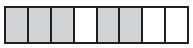Each Part is ________. ______ eighths
_____________

i) 1/8
ii) Five
iii) 5/8

Explanation:
There are 8 blocks in the rectangle out of which 5 parts are shaded. Each part of the rectangle is 1/8 and the name for the shaded part is Five Eights. The fraction of the shaded part is 5/8.

Question 3: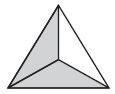Each Part is ________. ______ thirds
_____________

i) 1/3
ii) Two
iii) 2/3

Explanation:
Here we can see 3d form a triangle, each part is 1/3. Two sides are shaded in it so, the name for the shaded part is two thirds. The shaded part will be in the numerator and the total parts will be in the denominator. Hence the fraction form for the above fig is 2/3.

Question 4:Each Part is ________. ______ fourths
_____________

i) 1/4
ii) Three
iii) 3/4

Explanation:
The square is divided into 4 equal triangles. Each part of the square is 1/4 and there are 3 shaded triangles in the square box. The name for the shaded parts is three fourths i.e., 3/4

Question 5:
Four out of six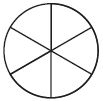Each Part is ______________

4/6

Explanation:
The circle is divided into 6 parts of which four parts are shaded. So, the numerator will be the shaded part and the denominator will be the total number of parts. So the answer is 4/6.

Question 6:
Eight out of eight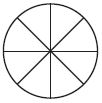Each Part is ______________

8/8

Explanation:
The fig above shows that the circle is equally divided into 8 parts out of which all the parts are shaded. So, the fraction form of the shaded part is 8/8.

Question 7:
Emma makes a poster for the school’s spring concert. She divides the poster into 8 equal parts. She uses two of the parts for the title. What fraction of the poster does Emma use for the title?
_________________

2/8

Explanation:
Given,
Emma divides the poster into 8 equal parts
She uses 2 parts for the title
So, the total number of parts will be in the denominator and the used parts will be in the numerator

Question 8:
Lucas makes a flag. It has 6 equal parts. Five of the parts are red. What fraction of the flag is red?
_________________

5/6

Explanation:
Luca makes a flag which has 6 equal parts, in which 5 of the parts are red.
The fraction of the flag is which is in red is?
5 parts of red will be in the numerator and the 6 will be in the denominator
So, the fraction of the flag red is 5/6.

Fractions of a Whole Lesson Check Page No 466

Question 1:
What fraction names the shaded part?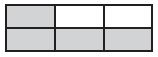Options:
i. 4/6
ii. 2/4
iii. 4/8
iv. 2/6

i (4/6)

Explanation:
The rectangle is divided into 6 equal parts. Among them, 4 parts of the rectangle are shaded. So the fraction name of the shaded part is 4/6.

Question 2:
What fraction names the shaded part?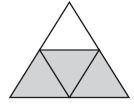Options:
i. One fourth
ii. One third
iii. Three fourths
iv. Four thirds

iii (Three fourths)

Explanation:
The above figure shows that the triangle is divided into 4 parts equally in which 3 parts are shaded. Therefore, the shaded part will be in the numerator and the total parts will be in the denominator. So, the fraction name of the shaded part is three fourths.

Question 3:
Sarah biked for 115 minutes last week. Jennie biked for 89 minutes last week. How many minutes in all did the girls bike?
Options:
i. 26 minutes
ii. 194 minutes
iii. 204 minutes
iv. 294 minutes

iii (204 minutes)

Explanation:
Given that,
Sarah biked for 115 minutes and,
Jennie biked for 89 minutes
To find:
How many minutes in all did the girls bike
To find the total minutes we need to add the bike ride time of both Sarah and Jennie
i.e., 115 + 89 = 204 minutes

Question 4:
Harrison made a building using 124 blocks. Greyson made a building using 78 blocks. How many more blocks did Harrison use than Greyson did?
Options:
i. 46
ii. 56
iii. 154
iv. 202

i (46)

Explanation:
i) Harrison made a building using 124 blocks
ii) Greyson made a building using 78 blocks
In order to know how many more blocks did Harrison use than Greyson did we need to subtract the blocks made by Harrison with Blocks made by Greyson
124 – 78 = 46

Question 5:
Von bought a bag of 24 dog treats. He gives his puppy 3 treats a day. How many days will the bag of dog treats last?
Options:
i. 3 days
ii. 6 days
iii. 8 days
iv. 21 days

iii (8 days)

Explanation:
Given,
Von bought a bag of 24 dog treats
He gives his puppy 3 treats a day
To find:
How many days will the bag of dog treats last
Here we have to divide no. of bag treats by puppy treats a day
24/3 = 8

Question 6:
How many students chose swimming?Options:
i. 5
ii. 10
iii. 20
iv. 25

iv (25)

Explanation:
From the figure, we can observe that there are 5 students who choose swimming. But each student is equal to 5 votes.
So, 5 × 5 = 25
Therefore the students who swimming are 25

Fractions on a Number Line Page No 471

Use fraction strips to help you complete the number line. Then locate and draw a point for the fraction.
Question 1:
1/3Question 2:
3/4Write the fraction that names the point.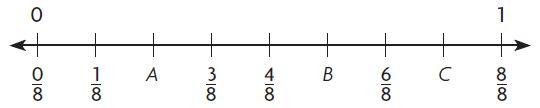Question 3:
Point A ________

Explanation:
The Number lies between 0 and 1 and each point is divided into 1/8. So, it starts from 0/8 to 8/8. Now we have to locate point A i.e., 2/8 because the number that lies after 1/8 is 2/8.

Question 4:
Point B ________

Explanation:
As we have discussed before point B lies between 4/8 and 6/8. So, the location of point B is 5/8.

Question 5:
Point C ________

Explanation:
The location of Point C lies between 6/8 and 8/8. Therefore the number between 6/8 and 8/8 is 7/8.

Problem Solving

Question 6:
Jade ran 6 times around her neighborhood to complete a total of 1 mile. How many times will she need to run to complete 5/6 of a mile?
_____

Explanation:
Given,
6 laps around the neighborhood = 1 mile
That means each lap = 1/6th of a mile
In order to complete 5/6 of a mile she has to run 5 times

Question 7:
A missing fraction on a number line is located exactly halfway between 3/6 and 5/6. What is the missing fraction?
_____

Explanation:
Given that the missing fraction on a number line is located between 3/6 and 5/6. The number that lies between 3 and 5 is 4. So, the missing fraction is 4/6.

Fractions on a Number Line Lesson Check Page No 472

Question 1:
Which fraction names point G on the number line?Options:
i. 1/4
ii. 2/4
iii. 4/4
iv. 4/1

i. (1/4)

Explanation:
The fraction on the number line lies between 0/4 and 4/4 i.e., 0 to 1. The location of point G lies between 0/4 and 2/4. The number between 0 and 2 is 1. Therefore the fraction of Point G is 1/4.

Question 2:
Which fraction names point R on the number line?Options:
i. 1/3
ii. 2/3
iii. 3/3
iv. 3/2

ii (2/3)

Explanation:
The number line starts from 0/3 and ends at 3/3. Point R is between 1/3 and 3/3. So, the fraction name of Point R on Number line is 2/3

Spiral Review

Question 3:
Each table in the cafeteria can seat 10 students. How many tables are needed to seat 40 students?
Options:
i. 10
ii. 8
iii. 5
iv. 4

iv (4)

Explanation:
Given,
1 table in the cafeteria can seat 10 students
The tables are needed to seat 40 students =?
1 —- 10 students
?—- 40 students
40/10 = 4
Therefore tables are needed to seat 40 students = 4

Question 4:
Which is an example of the Commutative Property of Multiplication?

Options:
i. 6 × 1 = 6 × 1
ii. 4 + 9 = 4 × 9
iii. 4 × 9 = 9 × 4
iv. 6 × 3 = 2 × 9

iii (4 × 9 = 9 × 4)

Explanation:
The multiplication rule for the commutative property is ab = ba. Therefore the answer is 4 × 9 = 9 × 4.

Question 5:
Pedro shaded part of a circle. Which fraction names the shaded part?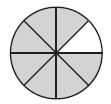Options:
i. 1/8
ii. 1/7
iii. 7/8
iv. 8/7

iii (7/8)

Explanation:
The circle is divided into 8 equal parts. In that 7 parts are shaded. So, the fraction name for the shaded part is 7/8.

Question 6:
Which is true?
Options:
i. 8 ÷ 1 = 8
ii. 8 ÷ 8 = 8
iii. 8 × 0 = 8
iv. 1 =  8 × 1

i (8 ÷ 1 = 8)

Explanation:
Any number divided by  1 is itself.
8/1 = 8
So the answer is 8 ÷ 1 = 8

Mid-Chapter Checkpoint Page No 473

Vocabulary

Choose the best term from the box to complete the sentence.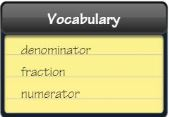Question 1:
A ____________ is a number that names part of a whole or part of a group.

Explanation:
The fraction is the one that divides the whole into equal parts or each part of the group.

Question 2:
The ___________ tells how many equal parts are in the whole or in the group.

Explanation:
The part of a fraction that lies below the line and which shows the total number of equal parts in the whole.

Concept And Skills

Write the number of equal parts. Then write the name for the parts.

Question 3: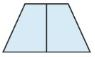Equal Parts: ________
Name: ____________

i. 2
ii. Halves

Explanation:
The trapezium is divided into two equal parts and the name for the parts is halves.

Question 4: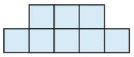Equal Parts: ________
Name: ____________

i. 8
ii. Eighths

Explanation:
From the above fig, we can observe that there are 8 equal parts that are in the shape of a square. The name for the parts of the figure is Eighths.

Question 5:Equal Parts: ________
Name: ____________

i. 4
ii. Fourths

Explanation:
The figure is the shape of a rectangle and it is divided into 4 right triangles. So, the equal parts of the rectangle are 4 and the name for the parts is fourths.

Write the number of equal parts in the whole. Then write the fraction that names the shaded part.

Question 6:Equal Parts ________

i. 3
ii. 1/3

Explanation:
A circle is divided equally into 3 parts and one part is shaded among them. So, the fraction that names the shaded part is 1/3

Question 7:Equal Parts ________

i. 6
ii. 1/6

Explanation:
The above figure is divided into 6 equal parts in the shape of the triangle. Only one part of the triangle is shaded among them. Therefore the fraction name for it is 1/6.

Question 8:Equal Parts ________

i. 4
ii. 1/4

Explanation:
Observe the above figure, there are 4 equal parts of the triangle in which one part is shaded. So, the fraction name for the shaded part is 1/4.

Mid-Chapter Checkpoint Page No 474

Write the fraction that names the point.Question 9:
Point A ______

Explanation:
The Number line lies from 0/6 and 6/6 in the fraction form. Point A lies between 0/6 and 3/6. So, the location of point A is 1/6

Question 10:
Point B ______

Explanation:
Point B lies between point A and 3/6. We know that Point A is 1/6. The number between 1/6 and 3/6 is 2/6. So, point B is 2/6.

Question 11:
Point C ______

Explanation:
Point C is located between 4/6 and 6/6. The number between 4 and 6 is 5. Therefore the fraction that names Point C is 5/6.

Question 12:
Jessica ordered a pizza. What fraction of the pizza has mushrooms? What fraction of the pizza does not have mushrooms?i. 2/8
ii. 6/8

Explanation:
Given,
Jessica ordered a pizza in which 2 parts of pizza has mushrooms
We need to write the fraction of the pizza that has mushrooms
Total Number of slices = 8
Pizza that has mushrooms = 2
Pizza that does not have mushrooms = 6
The fraction of the pizza that has mushrooms = 2/8
The fraction of the pizza that does not have mushrooms = 6/8

Question 13:
Which fraction names the shaded part?i. 8 Equal Parts
ii. 5/8

Explanation:
The figure shows that the rectangle is divided into 8 equal parts. Five parts are shaded in that rectangle. So, the fraction name for the parts that are shaded is 5/8.

Question 14:
Six friends share 3 oatmeal squares equally. How much of an oatmeal square does each friend get?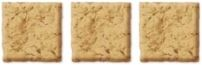Explanation:
Total no. of friends = 6
Number of oatmeal squares = 3
Total no. of friends will be in the denominator and the oatmeal in the numerator.
So the answer is 3/6 or 1/2.

Relate Fractions and Whole Numbers Page No 478

Make Connections Draw a model of the fraction or fraction greater than 1. Then write it as a whole number.

Question 12:
8/4 = _____

Question 13:
6/6 = _____

Question 14:
5/1 = _____

Question 15:
Jeff rode his bike around a bike trail that was 1/3 of a mile long. He rode around the trail 9 times. Write a fraction greater than 1 for the distance. How many miles did Jeff ride?

Explanation:
Given,
Jeff rode his bike around a bike trail that was 1/3 of a mile long
He rode around the trail 9 times
In order to know how many miles did Jeff Ride
We need to multiply 1/3 with 9
= 9 × 1/3
= 3 miles

Question 16:
What’s the Error? Andrea drew the number line below. She said that 9/8 and 1 are equal. Explain her errorThe error of Andrea is that she has located the point 1 on 9/8, but it must lie on 8/8.

Explanation:
Not Equal. Because 9/8 and 1 are not the same. Point 1 must be located on 8/8.

Question 17:
Each shape is 1 whole. Which numbers name the parts that are shaded? Mark all that apply.Options:
i. 4
ii. 6
iii. 26/6
iv. 24/6
v. 6/4

iv (24/6)

Explanation:
There are four circles and each circle is divided into six parts. All parts are shaded in each group. So, the total number of shaded parts is 24. The numerator consists of a number of shaded parts and the denominator contains a number of parts in each circle.
= 24/6

Relate Fractions and Whole Numbers Page No 479

Use the number line to find whether the two numbers are equal. Write equal or not equal.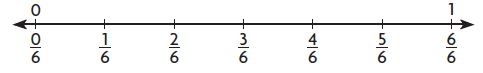Question 1:
0/6 and 1
____

Not equal

Explanation:
The points 0/6 and 1 does not lie on the same point. So, the numbers 0/6 and 1 are not equal

Question 2:
1 and 6/6
____

Equal

Explanation:
6/6 = 1
So 6/6 and 1 are equal

Question 3:
1/6 and 6/6
____

Not Equal

Explanation:
The points 1/6 and 6/6 does not lie on the same point. So, 1/6 and 6/6 are not equal.

Question 4:2 = ____

Explanation:
There are 2 circles and each circle is divided into two parts.
Total number of equal parts = 4
Number of circles =
So, 4/2 = 2

Question 5:4 = ____

Explanation:
From the above figure, we can see 4 circles which are divided 3 parts individually.
Number of Equal parts = 12
So, 12/3 = 4

Question 6: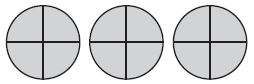3 = ____

Explanation:
Total Number of equal parts = 12
Equal parts in each circle = 4
Therefore, 12/4 = 3

Question 7: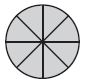1 = _____

Explanation:
A circle is divided into 8 equal parts and all are shaded. So, the fraction name for the shaded parts is 8/8.

Problem Solving

Question 8:
Rachel jogged along a trail that was 1/4 of a mile long. She jogged along the trail 8 times. How many miles did Rachel jog in all?
______ miles

Explanation:
Given,
Rachel jogged along a trail that was 1/4 of a mile long
And, She jogged along the trail 8 times
8 × 1/4 = 8/4 = 2
Therefore the answer is 2 miles.

Question 9:
Jon ran around a track that was 1/8 of a mile long. He ran around the track 24 times. How many miles did Jon run in all?
______ miles

Explanation:
Given that, Jon ran around a track that was 1/8 of a mile long
He ran around the track 24 times
24 × 1/8 = 24/8 = 3
So, the miles did Jon run in all is 3 miles

Relate Fractions and Whole Numbers Lesson Check Page No 480

Question 1:
Each shape is 1 whole. Which fraction greater than 1 names the parts that are shaded?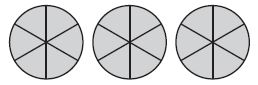Options:
i. 6/18
ii. 3/6
iii. 6/3
iv. 18/6

iv (18/6)

Explanation:
There are 3 circles which are divided into 6 parts = 18 parts
Each circle has 6 shaded parts
= 18/6

Question 2:
Each shape is 1 whole. Which fraction greater than 1 names the parts that are shaded?Options:
i. 8/2
ii. 16/8
iii. 8/16
iv. 2/8

ii (16/8)

Explanation:
There are two are which are divided into 8 parts = 8 × 2 = 16
Number of shaded parts in 1 circle = 8

Spiral Review

Question 3:
Tara has 598 pennies and 231 nickels. How many pennies and nickels does she have in all?
598 + 231

Options:
i. 719
ii. 729
iii. 819
iv. 829

iv (829)

Explanation:
598 + 231 = 829

Question 4:
Options:
i. 4
ii. 8
iii. 12
iv. 14

iii (12)

Explanation:
Given,
That means Kylie reads 2 times greater than Dylan
6 × 2 = 12

Question 5:
Alyssa divides a granola bar into halves. How many equal parts are there?
Options:
i. 2
ii. 3
iii. 4
iv. 6

i (2)

Explanation:
Given that, Alyssa divides a granola bar into halves
Halves mean 2. So there are 2 equal parts

Question 6:
There are 4 students in each small reading group. If there are 24 students in all, how many reading groups are there?
Options:
i. 5
ii. 6
iii. 7
iv. 8

ii (6)

Explanation:
Given that there are 4 students in each small reading group
To find:
how many reading groups are there for 24 students
So, Total number of students/ No. of students in each small reading group
i.e., 24/4 = 6

Fractions of a Group Page No 485

Write a fraction to name the shaded part of each group.
Question 1: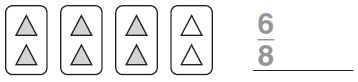______

Explanation:
There are 8 triangles in 4 blocks and 6 triangles are shaded among them. So, the fraction to name the shaded part is 6/8.

Question 2: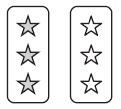______

Explanation:
In the above figure, we can observe that there are 6 stars in 2 groups and three stars are shaded. Shaded stars will be in the numerator and the total number of stars in the denominator. So, the answer is 3/6

Write a whole number and a fraction greater than 1 to name the part filled. Think: 1 container = 1

Question 3:______

i. 2
ii. 8/4

Explanation:
The figure shows that there are two containers and four circles are shaded. One container is the mirror image of another container. So, we can see 8 shaded circles. So, the fraction greater than 1 to name the part filled is 8/4.

Question 4: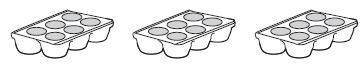______

i. 3
ii. 18/6

Explanation:
Here we can see three egg trays and all six parts are filled. By seeing the above figure we can understand that egg tray is the mirror image of other trays. So, we can see 18 parts filled. So, the fraction to the part filled is 18/6

Draw a quick picture. Then, write a fraction to name the shaded part of the group.

Question 5:
Draw 4 circles. Shade 2 circles.
_____

Question 6:
Draw 6 circles. Make 3 groups. Shade 1 group.
_____

Problem Solving

Question 7:
Brian has 3 basketball cards and 5 baseball cards. What fraction of Brian’s cards are baseball cards?
______

Explanation:
Given,
Brian has 3 basketball cards and 5 baseball cards
Total Number of cards = 5 + 3 = 8
The fraction of Brian’s cards are baseball cards =?
5/5+3 = 5/8

Question 8:
Sophia has 3 pink tulips and 3 white tulips. What fraction of Sophia’s tulips are pink?
______

Explanation:
Number of pink tulips Sophia has = 3
and No. of white tulips Sophia has = 3
Total Number of tulips = 3 + 3 = 6
To find the fraction of Sophia’s tulips are pink
= No. of pink tulips/Total No. of tulips
= 3/6

Fractions of a Group Lesson Check Page No 486

Question 1:
What fraction of the group is shaded?Options:
i. 5/3
ii. 5/8
iii. 3/5
iv. 3/8

ii (5/8)

Explanation:
There are a group of 8 circles and five are shaded in it. So, the fraction of the shaded group is 5/8

Question 2:
What fraction of the group is shaded?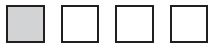Options:
i. 1/4
ii. 1/2
iii. 2/4
iv. 4/1

i. (1/4)

Explanation:
It is a group of four squares in which one block is shaded. So, the fraction of the shaded part is 1/4

Spiral Review

Question 3:
Which number sentence does the array represent?Options:
i. 4 × 7 = 28
ii. 3 × 8 = 24
iii. 3 × 7 = 21
iv. 3 × 6 = 18

iii (3 × 7 = 21)

Explanation:
Total Number of Rows = 3
Total Number of Columns = 7
Rows × Columns = 3 × 7 = 21

Question 4:
Juan has 436 baseball cards and 189 football cards. How many more baseball cards than football cards does Juan have?
Options:
i. 625
ii. 353
iii. 347
iv. 247

iv (247)

Explanation:
No. of baseball cards that Juan has = 436
No. of football cards that Juan has = 189
To know how many more baseball cards than football cards does Juan have,
we need to subtract No. of baseball cards and No. of football cards
= 436 – 189
= 247

Question 5:
Sydney bought 3 bottles of glitter. Each bottle of glitter costs $6. How much did Sydney spend in all on the bottles of glitter? Options: i.$24
ii. $18 iii.$12
iv. $9 Answer: ii ($18)

Explanation:
Given,
Sydney bought 3 bottles of glitter
Each bottle of glitter costs = $6 Cross multiplication method is applied here, 1 bottle —$6
3 bottles –?
3 × 6 = 18
Therefore, the cost of 3 bottles of glitter = \$18

Question 6:
262 + 119
Options:
i. 143
ii. 371
iii. 381
iv. 481

iii (381)

Explanation:
262 + 119 = 381

Find Part of a Group Using Unit Fractions Page No 491

Circle equal groups to solve. Count the number of items in 1 group.
Question 1:1/4 of 12 = ___

Explanation:
To solve the problem we can multiply the numerator with the whole number and divide by the denominator
= (1 × 12)/4
= 12/4
= 3

Question 2: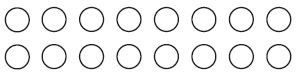1/8 of 16 = ___

Explanation:
In order to solve the problem we have to do product of 16 and 1/8
= 16 × 1/8
= 16/8
=2

Question 3: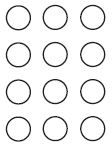1/3 of 12 = ___

Explanation:
The product of 1/3 and 12 is
(1 × 12)/3 = 12/3
We can cancel 12 in 3 table by 4 times
Therefore, 12/3 = 4

Question 4:1/3 of 9 = ___

Explanation:
We can label 1/3 and 9 as number and denominator of a whole number
= 9 × 1/3
= 3

Question 5: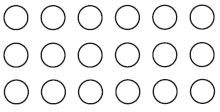1/6 of 18 = ___

Explanation:
The product of 1/6 and 18 is
(1 × 18)/3
= 6

Question 6: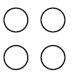1/2 of 4 = ___

Explanation:
It is a product of a fraction and whole numbers
The numerator is 1 and 4 and denominator is 2
1 × 4/2
=2

Problem Solving

Question 7:
Marco drew 24 pictures. He drew 1/6 of them in art class. How many pictures did Marco draw in art class?
______ Pictures

Explanation:
Given that Marco drew 24 picture
He drew 1/6 of them in art class
In order to know the pictures did Marco draw in art class
We have to multiply 1/6 with 24
we get,
24 ×1/6
= 24/6
= 4

Question 8:
Caroline has 16 marbles. One-eighth of them are blue. How many of Caroline’s marbles are blue?
______ Marbles

Explanation:
Number of marbles that Caroline has = 16
In that 1/8 are blue

To find:
Number of Caroline’s marbles are blue
So we have to do product of 16 and 1/8
1/8 × 16
16/8 = 2

Therefore Number of Caroline’s marbles are blue is 2

Find Part of a Group Using Unit Fractions Lesson Check Page No 492

Question 1:
Ms. Davis made 12 blankets for her grandchildren. One third of the blankets are blue. How many blue blankets did she make?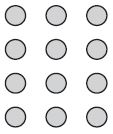Options:
i. 3
ii. 4
iii. 9
iv. 12

ii (4)

Explanation:
Number of blankets made for her grandchildren = 12
1/3rd of the blankets are blue
In order to know the count of blue blankets
Multiply 12 with 1/3
= (12 ×1)/3
= 4

Question 2:
Jackson mowed 16 lawns. One fourth of the lawns are on Main Street. How many lawns on Main Street did Jackson mow?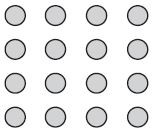Options:
i. 4
ii. 6
iii. 8
iv. 12

i (4)

Explanation:
Given that Jackson mowed 16 lawns
One-fourth of the lawns are on Main Street

To find:
How many lawns on Main Street did Jackson mow?
Product of 16 and 1/4
= 16 × 1/4
= 4

Question 3:
Find the difference.
509 – 175
Options:
i. 334
ii. 374
iii. 434
iv. 474

i.  (334)

Explanation:
The difference between the 1st number and 2nd number is
509 – 175 = 334

Question 4:
Find the quotient.
6)54
Options:
i. 6
ii. 7
iii. 8
iv. 9

iv. (9)

Explanation:
Divide 54 by 6
54/6 = 9
So, the remainder is 0 and quotient is 9

Question 5:
There are 226 pets entered in the pet show. What is 226 rounded to the nearest hundred?
Options:
i. 200
ii. 220
iii. 300
iv. 400

i. (200)

Explanation:
The word form of 226 is two hundred and twenty-six.
The number which is near to 226 is 200. Because the number is less than 250.
So, the nearest hundred to 226 is 200.

Question 6:
Ladonne made 36 muffins. She put the same number of muffins on each of 4 plates. How many muffins did she put on each plate?
Options:
i. 3
ii. 6
iii. 9
iv. 12

iii (9)

Explanation:
Given,
She put the same no. of muffins on each of 4 plates
No. of muffins on 4 plates/ Total No. of muffins
= 36/4
= 9

Problem Solving – Find the Whole Group Using Unit Fractions Page No 497

Draw a quick picture to solve.

Question 1:
Katrina has 2 blue ribbons for her hair. One fourth of all her ribbons are blue. How many ribbons does Katrina have in all?____ ribbons

8 ribbons

Explanation:
Given,
Katrina has 2 blue ribbons for her hair
1/4th of all ribbons are blue
To know how many ribbons that Katrina has,
we have to divide the number of blue ribbons by 1/4th of all ribbons are blue
we get, 2 ÷ 1/4 = (2 × 4)/1
= 2 × 4 = 8
Therefore, the answer to the above question is 8 ribbons.

Question 2:
One-eighth of Tony’s books are mystery books. He has 3 mystery books. How many books does Tony have in all?
______ Books

24 Books

Explanation:
Given that, Tony has 3 mystery books
Out of which 1/8th of tony’s books are mystery books
So, to find how many books does Tony have in all
Divide 3 by 1/8, we get
3 ÷ 1/8
(3 ×8)/1 = 24

Question 3:
Brianna has 4 pink bracelets. One-third of all her bracelets are pink. How many bracelets does Brianna have?
______ Bracelets

12 Bracelets

Explanation:
No. of pink bracelets that Brianna has = 4
1/3rd of all her bracelets are pink
Divide No. of pink bracelets by 1/3rd of all her pink bracelets
we get,
4 ÷ 1/3
4 × 3 = 12
So, the answer is 12 bracelets

Question 4:
Ramal filled 3 pages in a stamp album. This is one sixth of the pages in the album. How many pages are there in Ramal’s stamp album?
______ pages

18 pages

Explanation:
Given,
Ramal filled 3 pages in a stamp album
one-sixth of the pages in the album
3 ÷ 1/6 = 3 × 6 = 18 pages

Question 5:
Jeff helped repair one half of the bicycles in a bike shop last week. If Jeff worked on 5 bicycles, how many bicycles did the shop repair in all last week?
______ bicycles

10 bicycles

Explanation:
Jeff helped repair 1/2 of the bicycles in a bike shop last week
Jeff worked on 5 bicycles
5 divided by 1/2
5 × 2/1 = 10 bicycles

Question 6:
Layla collects postcards. She has 7 postcards from Europe. Her postcards from Europe are one third of her total collection. How many postcards in all does Layla have?
______ postcards

21 postcards

Explanation:
Layla collects postcards in which 7 postcards are from Europe
Postcards from Europe of her total collection = 1/3
7 divided by 1/3
we get, 7 × 3 = 21
Therefore, the postcards in all do Layla has 21 postcards

Find the Whole Group Using Unit Fractions Lesson Check Page No 498

Question 1:
A zoo has 2 male lions. One-sixth of the lions are male lions. How many lions are there at the zoo?
Options:
i. 2
ii. 6
iii. 8
iv. 12

iv (12)

Explanation:
Male lions in the Zoo = 2
1/6th of the lions are male in the zoo
2 ÷ 1/6
= 2 × 6
= 12

Question 2:
Max has 5 red model cars. One-third of his model cars are red. How many model cars does Max have?
Options:
i. 15
ii. 12
iii. 10
iv. 8

i.  (15)

Explanation:
Given,
Max has 5 red model cars
1/3rd of the cars are red
So we need to divide 5 by 1/3,
we get 3 × 5 = 15

Spiral Review

Question 3:
There are 382 trees in the local park. What is the number of trees rounded to the nearest hundred?
Options:
i. 300
ii. 380
iii. 400
iv. 500

iii. (400)

Explanation:
Given that there are 382 trees in the local park.
This is nearer to the number 400. So the round figure of 382 is 400

Question 4:
The Jones family is driving 458 miles on their vacation. So far, they have driven 267 miles. How many miles do they have left to drive?
458 – 267
Options:
i. 191
ii. 201
iii. 211
iv. 291

i.  (191)

Explanation:
Jones family is driving 458 miles on their vacation
They have driven 267 miles
In this, we have to subtract 458 with 267,
we get, 191

Question 5:
Ken has 6 different colors of marbles. He has 9 marbles of each color. How many marbles does Ken have in all?
Options:
i. 15
ii. 45
iii. 54
iv. 63

iii (54)

Explanation:
Given,
Ken has 6 different colors of marbles
Ken has 9 marbles of each color
To know the total number of marbles multiply different colors of marbles with each marble color
= 6 × 9 = 54

Question 6:
Eight friends share two pizzas equally. How much of a pizza does each friend get?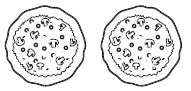Options:
i. 8 halves
ii. 4 eighths
iii. 2 sixths
iv. 2 eighths

iv (2 eighths)

Explanation:
There are 2 pizzas and eight friends need to share those pizzas
so, we have 2 by 8
Each friend gets 2/8 and the fraction name is 2 eighths

Chapter 8 Understanding Fractions Review Test – Page No 499

Review/Test

Question 1:
Each shape is divided into equal parts. Select the shapes that show thirds. Mark all that apply.

Options:
i.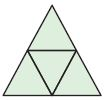ii.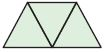iii.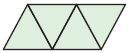iv.Explanation: From the given figures we can observe that there are thirds in Fig ii & iv.

Question 2:
What fraction names the shaded part of the shape?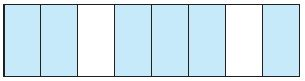Options:
i. 8 sixths
ii. 8 eighths
iii. 6 eighths
iv. 2 sixths

iii (6 eighths)

Explanation:
A rectangle is divided into a group of eight parts and 6 parts are shaded. The fraction name of the shaded parts is 6 eighths.

Question 3:
Omar shaded a model to show the part of the lawn that he finished mowing. What fraction names the shaded part? Explain how you know how to write the fraction.Explanation:
From the figure, we can see that there are eight triangles and only one part is shaded. So, the fraction of the shaded part is 1/8.

No. of Shaded parts must be given in the numerator and the number of parts or whole group will be in the denominator.

Chapter 8 Understanding Fractions Review Test – Page No 500

Question 4:
What fraction names point A on the number line?Point A ___

Explanation:
A number line is shown in the figure above,
Each point is equal to 1/6
The point is located after 0/6. So, Point A is 1/6.

Question 5:
Jamal folded this piece of paper into equal parts. Circle the word that makes the sentence true.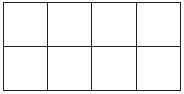The Paper is folded into

Options:
i. Sixths
ii. Eighths
iii. Fourths

Explanation:
From the figure, we can see that the paper is divided into 8 equal parts. So, the paper is folded into eighths.

Question 6:
Caleb took 18 photos at the zoo. One sixth of his photos are of giraffes. How many of Caleb’s photos are of giraffes?
_______ photos

Explanation:
Given that,
Caleb took 18 photos at the zoo
1/6th of the photos are giraffes
To know the no. of Caleb’s photos are of giraffes
Simplify 18 and 1/6
18/6 = 3

Question 7:
Three teachers share 2 packs of paper equally.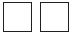How much paper does each teacher get? Mark all that apply.
Options:
i. 3 halves of a pack
ii. 2 thirds of a pack
iii. 3 sixths of a pack
iv. 1 half of a pack
v. 1 third of a pack

ii (2 thirds of a pack)

Explanation:
No. of teachers = 3
No. of Packs of paper = 2
3 teachers should share 2 packs of paper
i.e., No. of Paper Packs/ No. of teachers = 2/3
The fraction name is 2 thirds of a pack

Chapter 8 Understanding Fractions Review Test – Page No 501

Question 8:Select one number from each column to show the part of the design that Lilly shaded.i. Numerator 1
ii. Denominator 4
iii. Numerator 5

Explanation:
The task is to observe the figure and identify the number from each column i.e., Numerator and Denominator Column. Lilly has Shaded the 1st column 1st block i.e, 1, Next 2nd Column 2nd block is shaded so 4, and at last 1st column 3rd block is shaded so numerator 5.

Question 9:
Marcus baked a loaf of banana bread for a party. He cut the loaf into equal size pieces. At the end of the party, there were 6 pieces left. Explain how you can find the number of pieces in the whole loaf if Marcus told you that 1/3 of the loaf was left. Use a drawing to show your work.
____ pieces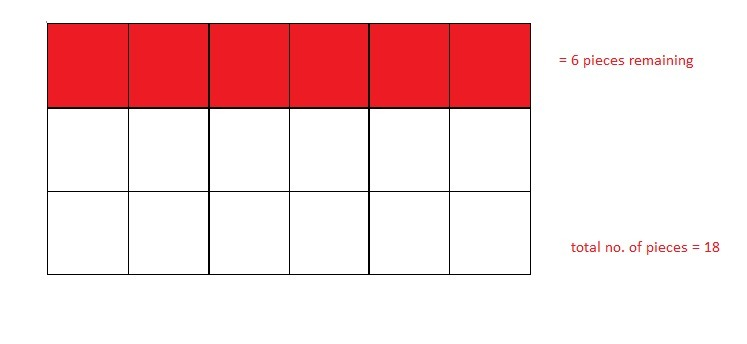Explanation:
Given,
Marcus baked a loaf of banana bread for a party. He cut the loaf into equal size pieces
At the end of the party, there were 6 pieces left.
Marcus told you that 1/3 of the loaf was left
To find:
Number of pieces in the whole loaf = x
1/3 × x = 6
x = 6 × 3
x = 18
So, the total number of pieces in the whole loaf is 18

Chapter 8 Understanding Fractions Review Test – Page No 502

Question 10:
The model shows one whole. What fraction of the model is NOT shaded?____

Explanation:
The square is divided to 4 equal triangles. In that two parts are shaded and two parts are not shaded.
Thus the fraction for the non shaded part is 2/4

Question 11:
Together, Amy and Thea make up 1/4 of the midfielders on the soccer team. How many midfielders are on the team? Show your work.
_____ midfielders

Explanation:
1/4 of the midfielders on the soccer team represents 2 midfielders
If we divide no. of midfielders into 4 equal groups, then each group will have 2 midfielders
1/4 × x = 2
x = 4 × 2
x = 8
Thus 8 midfielders are on the team

Question 12:
Six friends share 4 apples equally. How much apple does each friend get?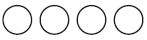Question 13:
Each shape is 1 whole.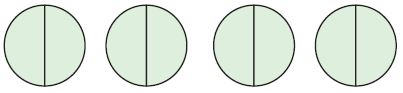For numbers, 13a–13e, choose Yes or No to show whether the number names the parts that are shaded.

a. 4
i. Yes
ii. No

i. Yes

b. 8
i. Yes
ii. No

i. Yes

c. 8/2
i. Yes
ii. No

ii. No

d. 8/4
i. Yes
ii. No

i. Yes

e. 2/8
i. Yes
ii. No

ii. No

Chapter 8 Understanding Fractions Review Test – Page No 503

Question 14:
Alex has 3 baseballs. He brings 2 baseballs to school. What fraction of his baseballs does Alex bring to school?
____

Explanation:
Total Number of baseballs that Alex have = 3
He brings 2 baseballs to school
The fraction of baseballs that brings to school = No. of baseballs brings to school/total no. of baseballs
= 2/3

Question 15:
Part A
Janeen used 16 pieces of fruit to make her salad. If 1/4 of the fruits were peaches, how many peaches did she use? Make a drawing to show your work.
____ peaches

No. of pieces Janeen used to make her fruit salad = 16
In that 1/4 of the fruits were peaches
how many peaches did she use is?
Multiply No. of fruit pieces with 1/4 of the fruits were peaches
we get,
16 × 1/4
= 16/4
= 4

Part B
Nicole used 24 pieces of fruit. If 1/6 of them were peaches, how many peaches in all did Janeen and Nicole use to make their fruit salads? Explain how you found your answer.
____ peaches

Explanation:
No. of peaches Janeen used in her fruit salad = 4
Total number of fruit pieces Nicole used = 24
If 1/6 of them were peaches
24 × 1/6 = 4
To know total no. of peaches that Janeen and Nicole used
We have to add the number of peaches in the fruit salad of Janeen and Nicole
= 4 + 4
= 8

Question 16:
There are 8 rows of chairs in the auditorium. Three of the rows are empty. What fraction of the rows are empty?
_____ rows

Explanation:
Given: Total Number of rows of chairs in the auditorium = 8
In that three rows are empty
The fraction of the rows that are empty is 3/8

Chapter 8 Understanding Fractions Review Test – Page No 504

Question 17:
Tara ran 3 laps around her neighborhood for a total of 1 mile yesterday. Today she wants to run 2/3 of a mile. How many laps will she need to run around her neighborhood?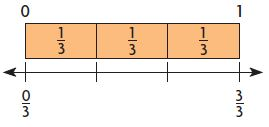____ laps

Explanation:
Given that,
Tara ran 3 laps around her neighborhood for a total of 1 mile
she wants to run 2/3 of a mile
1 mile —- 3 laps
2/3 mile — x
1 × x = (3 ×2)/3
x = 6/3
x = 2
Thus it takes 2 laps to run around her neighborhood

Question 18:
Gary painted some shapes.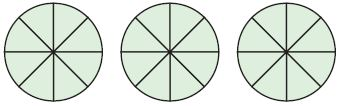Select one number from each column to show a fraction greater than 1 that names the parts Gary painted._____

Question 19:
Angelo rode his bike around a bike trail that was 1/4 of a mile long. He rode his bike around the trail 8 times. Angelo says he rode a total of 8/4 miles. Teresa says he is wrong and that he actually rode 2 miles. Who is correct? Use words and drawings to explain how you know.
_____